• 应用切比雪夫滤波器，设计了带通滤波器 function y=bandp(x,f1,f3,fsl,fsh,rp,rs,Fs) %带通滤波 %使用注意事项：通带或阻带的截止频率与采样率的选取范围是不能超过采样率的一半 %即，f1,f3,fs1,fsh,的值小于 Fs/2 %...
• hbchifan的回答： % 用切比雪夫最佳一致逼近设计线性相位FIR带通滤波器； %信号为0.5hz, 0.9hz, 1.1hz和1.5hz的正统信号叠加组成 %通带为[0.9,1.1] %频谱分辨率与信号实际长度N成正比 clear all; f1=0.5;f2=0.9;f3=...
盘国英谭婷的回答：你自己整合吧，我没时间帮你整合，我给你提供一些程序：绝对正确的代码：程序1：fs=22050;%语音信号采样频率为22050x1=wavread('windowscriticalstop.wav');%读取语音信号的数据，赋给变量x1sound(x1,22050);%播放语音信号y1=fft(x1,1024);%对信号做1024点fft变换f=fs*(0:511)/1024;figure(1)plot(x1)%做原始语音信号的时域图形title('原始语音信号');xlabel('timen');ylabel('fuzhin');figure(2)freqz(x1)%绘制原始语音信号的频率响应图title('频率响应图')figure(3)subplot(2,1,1);plot(abs(y1(1:512)))%做原始语音信号的fft频谱图title('原始语音信号fft频谱')subplot(2,1,2);plot(f,abs(y1(1:512)));title('原始语音信号频谱')xlabel('hz');ylabel('fuzhi');程序2：fs=22050;%语音信号采样频率为22050x1=wavread('windowscriticalstop.wav');%读取语音信号的数据，赋给变量x1t=0:1/22050:(size(x1)-1)/22050;y1=fft(x1,1024);%对信号做1024点fft变换f=fs*(0:511)/1024;x2=randn(1,length(x1));%产生一与x长度一致的随机信号sound(x2,22050);figure(1)plot(x2)%做原始语音信号的时域图形title('高斯随机噪声');xlabel('timen');ylabel('fuzhin');randn('state',0);m=randn(size(x1));x2=0.1*m+x1;sound(x2,22050);%播放加噪声后的语音信号y2=fft(x2,1024);figure(2)plot(t,x2)title('加噪后的语音信号');xlabel('timen');ylabel('fuzhin');figure(3)subplot(2,1,1);plot(f,abs(y2(1:512)));title('原始语音信号频谱');xlabel('hz');ylabel('fuzhi');subplot(2,1,2);plot(f,abs(y2(1:512)));title('加噪后的语音信号频谱');xlabel('hz');ylabel('fuzhi');根据以上代码，你可以修改下面有错误的代码程序3:双线性变换法设计butterworth滤波器fs=22050;x1=wavread('h:\课程设计2\shuzi.wav');t=0:1/22050:(size(x1)-1)/22050;au=0.03;d=[au*cos(2*pi*5000*t)]';x2=x1+d;wp=0.25*pi;ws=0.3*pi;rp=1;rs=15;fs=22050;ts=1/fs;wp1=2/ts*tan(wp/2);%将模拟指标转换成数字指标ws1=2/ts*tan(ws/2);[n,wn]=buttord(wp1,ws1,rp,rs,'s');%选择滤波器的最小阶数[z,p,k]=buttap(n);%创建butterworth模拟滤波器[bap,aap]=zp2tf(z,p,k);[b,a]=lp2lp(bap,aap,wn);[bz,az]=bilinear(b,a,fs);%用双线性变换法实现模拟滤波器到数字滤波器的转换[h,w]=freqz(bz,az);%绘制频率响应曲线figure(1)plot(w*fs/(2*pi),abs(h))gridxlabel('频率／hz')ylabel('频率响应幅度')title('butterworth')f1=filter(bz,az,x2);figure(2)subplot(2,1,1)plot(t,x2)%画出滤波前的时域图title('滤波前的时域波形');subplot(2,1,2)plot(t,f1);%画出滤波后的时域图title('滤波后的时域波形');sound(f1,22050);%播放滤波后的信号f0=fft(f1,1024);f=fs*(0:511)/1024;figure(3)y2=fft(x2,1024);subplot(2,1,1);plot(f,abs(y2(1:512)));%画出滤波前的频谱图title('滤波前的频谱')xlabel('hz');ylabel('fuzhi');subplot(2,1,2)f1=plot(f,abs(f0(1:512)));%画出滤波后的频谱图title('滤波后的频谱')xlabel('hz');ylabel('fuzhi');程序4:窗函数法设计滤波器：fs=22050;x1=wavread('h:\课程设计2\shuzi.wav');t=0:1/22050:(size(x1)-1)/22050;au=0.03;d=[au*cos(2*pi*5000*t)]';x2=x1+d;wp=0.25*pi;ws=0.3*pi;wdelta=ws-wp;n=ceil(6.6*pi/wdelta);%取整wn=(0.2+0.3)*pi/2;b=fir1(n,wn/pi,hamming(n+1));%选择窗函数，并归一化截止频率figure(1)freqz(b,1,512)f2=filter(bz,az,x2)figure(2)subplot(2,1,1)plot(t,x2)title('滤波前的时域波形');subplot(2,1,2)plot(t,f2);title('滤波后的时域波形');sound(f2,22050);%播放滤波后的语音信号f0=fft(f2,1024);f=fs*(0:511)/1024;figure(3)y2=fft(x2,1024);subplot(2,1,1);plot(f,abs(y2(1:512)));title('滤波前的频谱')xlabel('hz');ylabel('fuzhi');subplot(2,1,2)f2=plot(f,abs(f0(1:512)));title('滤波后的频谱')xlabel('hz');ylabel('fuzhi');hbchifan的回答：% 用切比雪夫最佳一致逼近设计线性相位FIR带通滤波器；%信号为0.5hz, 0.9hz, 1.1hz和1.5hz的正统信号叠加组成%通带为[0.9,1.1]%频谱分辨率与信号实际长度N成正比clear all;f1=0.5;f2=0.9;f3=1.1;f4=1.5;t=0:1203;N=length(t);fs=10;M=512;x1=sin(2*pi*(f1/fs)*t)+sin(2*pi*(f2/fs)*t)+sin(2*pi*(f3/fs)*t)+sin(2*pi*(f4/fs)*t);figure(1);subplot(211);plot(t,x1);title('原信号');y=fft(x1);f=(0:1/N:1/2-1/N)*fs;subplot(212);plot(f,abs(y(1:N/2)));grid;xlabel('hz');%处理前频谱wc1=2*f2/fs;wc2=2*f3/fs;wc3=2*f4/fs;%归一化角频率，用于下面的f1f1=[0 wc1-0.05 wc1 wc2 wc2+0.05 1];A=[0 0 1 1 0 0];%设置带通或带阻，1为带通，0为带阻weigh=[1 1 1 ];%设置通带和阻带的权重b=remez(60,f1,A,weigh);%传函分子h1=freqz(b,1,M);%幅频特性figure(2)f=(0:1/M:1-1/M)*fs/2;subplot(211);plot(f,abs(h1));grid;title('带通');x2=filter(b,1,x1);S1=fft(x2);f=(0:1/N:1/2-1/N)*fs;subplot(212);plot(f,abs(S1(1:N/2)));grid;xlabel('hz');%处理后频谱
展开全文• 用基本数学方程设计带通滤波器的程序，对没有信号处理工具箱的... 三角正弦函数和汉明窗方程用于创建带通滤波器。 通过改变中心频率、带宽参数，滤波器可以定制到所需的频率。 可以参考相同的程序来创建 LPF 和 HPF。
• 看到一个带通滤波器的设计如下，开始研究是如何设计的 代码： band_low = 0.3; band_high = 1.2; fs_origin = 25; hd_band = design(fdesign.bandpass('N,F3dB1,F3dB2',4,band_low,band_high,fs_origin),'...
写在前面的话：

由滤波器系数得到传输函数：

使用matlab fdatool设计滤波器，得到幅频响应，得到滤波器系数，由系数如何得到滤波器的参数？

看到一个带通滤波器的设计如下，开始研究是如何设计的

带通滤波器设计matlab代码：

band_low = 0.3;
band_high = 1.2;
fs_origin = 25;
hd_band = design(fdesign.bandpass('N,F3dB1,F3dB2',4,band_low,band_high,fs_origin),'butter'); %带通滤波器
%fvtool(hd_band); % view
measure(hd_band) %Measure the frequency response characteristics of the filter
%filtered = filter(hd_band,x);  % 滤波器的使用，输入数组x,输出数组filtered

查看官网MathWorks,找到关于滤波器的设计design,带通滤波器的设计fdesign.bandpass

The fdesign.bandpass function returns a bandpass filter design specification object that contains specifications for a filter such as passband frequency, stopband frequency, passband ripple, and filter order. Use the design function to design the filter from the filter design specifications object.

本处只解析此处滤波器的设计参数，若查看更多，移至官网MathWorks

N:Filter order for FIR filters. Or both the numerator and denominator orders for IIR filters when Na and Nb are not provided. Specified using FilterOrder. 关于滤波其的阶数
F3dB1:Frequency of the 3 dB point below the passband value for the first cutoff, specified in normalized frequency units. Applies to IIR filters.
F3dB2:Frequency of the 3 dB point below the passband value for the second cutoff, specified in normalized frequency units. Applies to IIR filters.
Fs bandpassSpecs = fdesign.bandpass(___,Fs) provides the sample rate in Hz of the signal to be filtered. Fs must be specified as a scalar trailing the other numerical values provided. In this case, all frequencies in the specifications are in Hz as well.
上面这句话解释了传入采样频率的必要性。

'N,F3dB1,F3dB2'
butter
以上参数的设置使用与IIR butter

fdesign.bandpass('N,F3dB1,F3dB2',4,band_low,band_high,fs_origin)
show:
bandpass (具有属性):

Response: 'Bandpass'
Specification: 'N,F3dB1,F3dB2'
Description: {3×1 cell}
NormalizedFrequency: 0
Fs: 25
FilterOrder: 4
F3dB1: 0.3000
F3dB2: 1.2000

fvtool(hd_band)% 查看幅频特性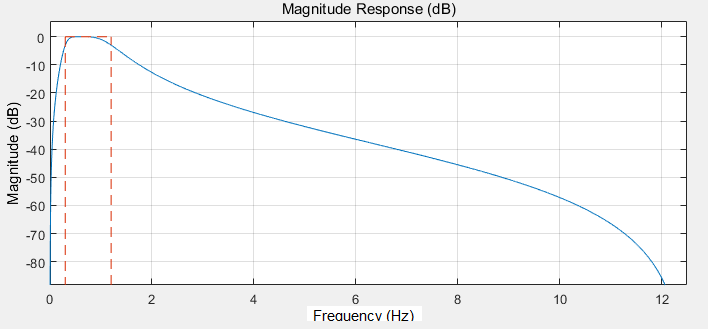measure(hd_band) %Measure the frequency response characteristics of the filter

Sample Rate             : 25 Hz
First Stopband Edge     : Unknown
First 6-dB Point        : 250.7041 mHz
First 3-dB Point        : 300 mHz
First Passband Edge     : Unknown
Second Passband Edge    : Unknown
Second 3-dB Point       : 1.2 Hz
Second 6-dB Point       : 1.4315 Hz
Second Stopband Edge    : Unknown
First Stopband Atten.   : Unknown
Passband Ripple         : Unknown
Second Stopband Atten.  : Unknown
First Transition Width  : Unknown
Second Transition Width : Unknown  

使用fdatool同样实现此滤波器的设计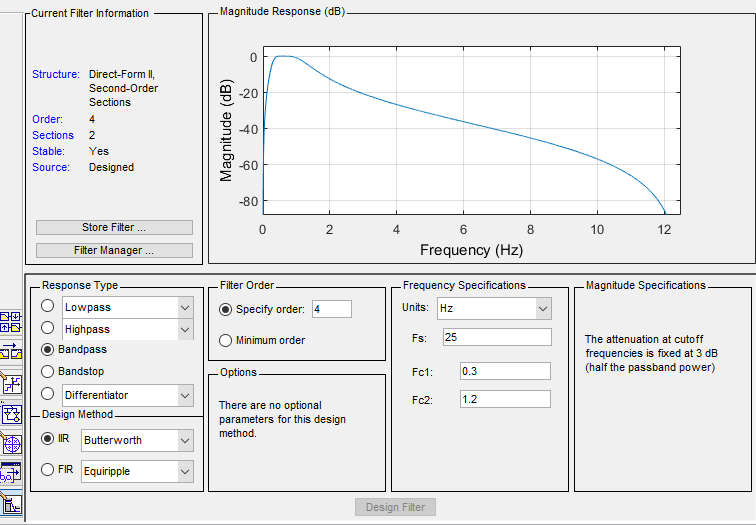设计的滤波器通过File-Export导出到工作区；

也可以在analysis中查看器信息，比如系数、幅频、相频特性。比如查看analysis中点击Filter Information中可以看到fdesign使用的内容：'N,F3dB1,F3dB2'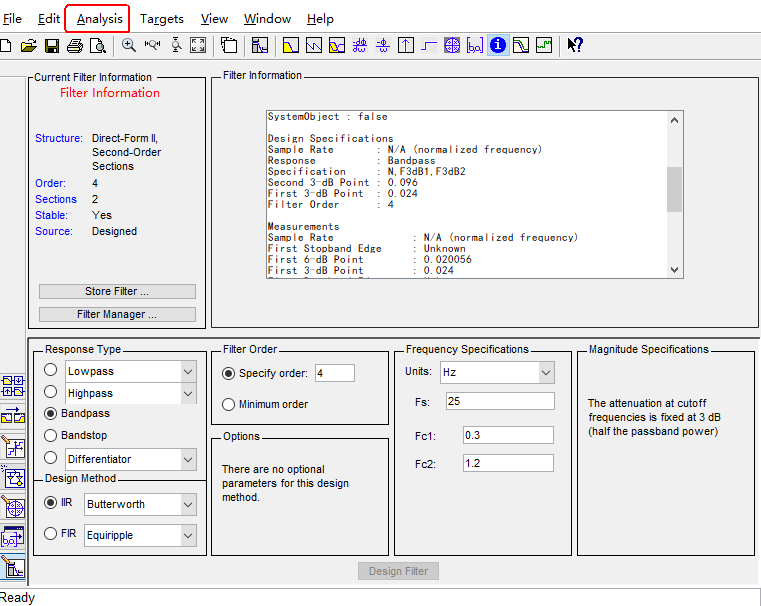完成了带通滤波器的设计，就在考虑如何转化为C语言代码，这里有个网站可以进行转化，设计滤波器转为C语言实现，但是结果并没有达到我想要的要求，我的目的是怎样将fdatool中设计的滤波器系数中得到其对应的传递函数H(Z)，有了传递函数，就可以自己写C语言实现了。

在上面fdatool设计的滤波器中，两种方法查看设计的滤波器，任意一种都可以；

或者采用如下图操作，从左到右三个按键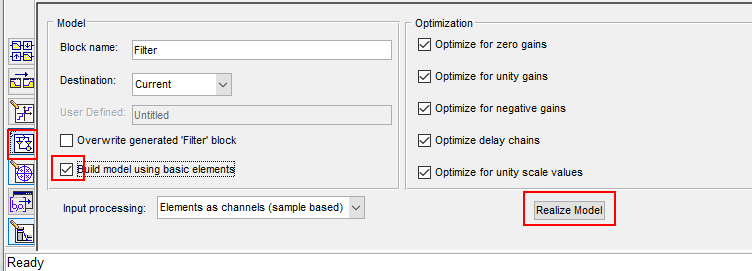双击可以看到滤波器的内部结构，如图显示为两个二阶滤波器级联：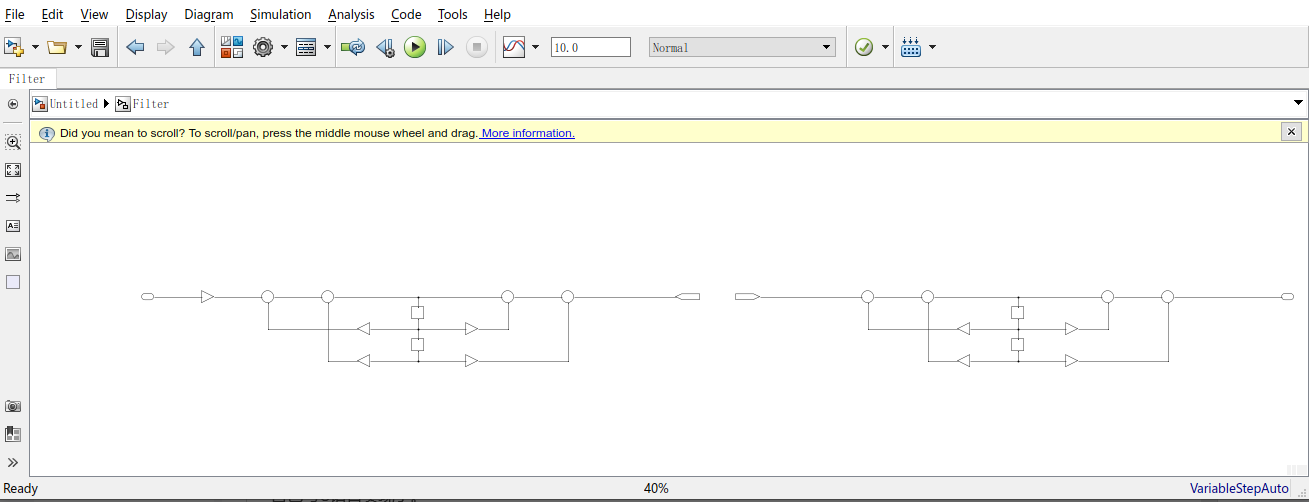放大其中一个滤波器如下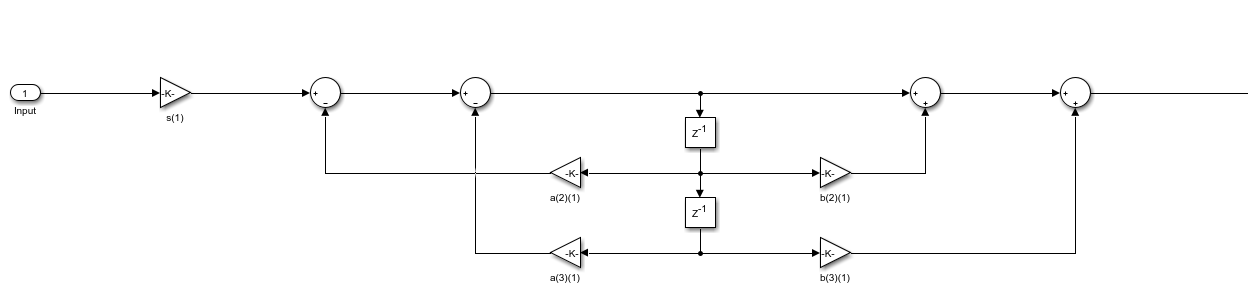简单作了标记如下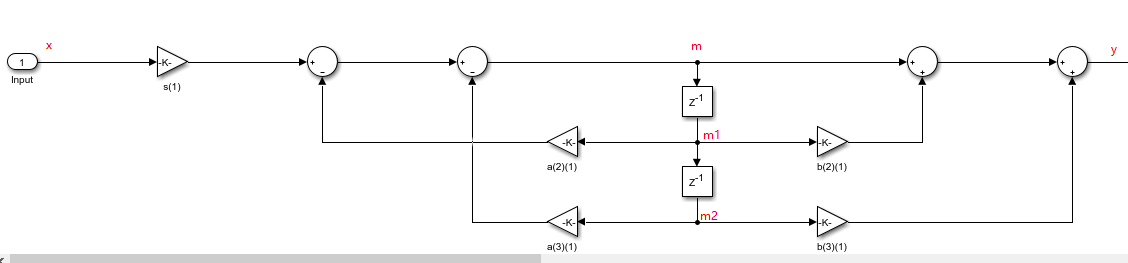并给出其对应的C语言实现，此代码无法直接运行只是提取了一部分带通滤波器实现部分

/* IIR fourth-order filter coefficients */
typedef struct
{
float(*a);
float(*b);
float* gain;
} IIR_ORDER4_COEFF_T;

/* Save the in-process' data of the second-order filter */
typedef struct
{
float m1;
float m2;
} FILTER_ORDER2_T;

/*三轴信号处理前的滤波：四阶带通(0.3-1.2)Hz)滤波，由两个二阶滤波级联起来*/
float a_raw = {
{ 1.00, (float)-1.9175958145710057, (float)0.9246554650412394 },    //第一级二阶滤波，分母
{ 1.00, (float)-1.7227109782351659, (float)0.7854234338937474 }    //第二级二阶滤波，分母
};//分母
float b_raw = {
{ 1.00, (float)0.00, (float)-1.00 },    //第一级二阶滤波，分子
{ 1.00, (float)0.00, (float)-1.00 }    //第二级二阶滤波，分子
};//分子
float gain_raw = { (float)0.1048485643154691/*第一级增益*/, (float)0.1048485643154691/*第二级增益*/ };

/******************************************************************************/
/** Start, initialize the parameters
* @param[in]
*
* @return
*
*//*
* History        :
* 1.Date         : 2020/8/28
*   Author       :
*   Modification : Created function

*******************************************************************************/
void StartSwim(void)
{
/* signal filter parameter initialization */
IIR_Raw.a = a_raw;
IIR_Raw.b = b_raw;
IIR_Raw.gain = gain_raw;
}

/*
x: input
m1:保存中间滤波器数据
m2:保存中间滤波器数据
a:滤波器系数
b:滤波器系数
gain:增益，对于只支持定点小数运算的，需要把增益分配到每个二阶IIR滤波器的系数中，使得每次中间的结果都不溢出，即使其频率响应的最大值最接近0dB
*/
float filterOrder2(float* x, float* m_1, float* m_2, float* a, float* b, float* gain){
float y, m;
//计算没有增益的滤波输出，存于y_
m = *x - a * *m_1 - a * *m_2;    //求当前m，同时作为求y_的中间步骤
y = m + b * *m_1 + b * *m_2;
//更新*m_1和*m_2
*m_2 = *m_1;
*m_1 = m;
//返回带增益的滤波输出
return y* *gain;
}

/******************************************************************************/
/** filter: Fourth-order filter, direct type II
* @param[in]
* filter_state	:Store the middle state of the filter
* @return
*
*//*
* History        :
* 1.Date         : 2020/8/28
*   Author       :
*   Modification : Created function

*******************************************************************************/
float FilterOrder4(float data, FILTER_ORDER4_T* filter_state, IIR_ORDER4_COEFF_T* IIR_coeffs)
{
float data_filtered;

filter_state->first_order2_out = filterOrder2(&data, &filter_state->first_order2.m1, &filter_state->first_order2.m2, IIR_coeffs->a, IIR_coeffs->b, IIR_coeffs->gain);  //第一级二阶滤波
data_filtered = filterOrder2(&filter_state->first_order2_out, &filter_state->second_order2.m1, &filter_state->second_order2.m2, IIR_coeffs->a, IIR_coeffs->b, &IIR_coeffs->gain);  //第二级二阶滤波

return data_filtered;
}

/******************************************************************************/
/** Band-pass filtering for the norm data: 0.25-2Hz
* @param[in] x:norm data
*
* @return filtered data
*
*//*
* History        :
* 1.Date         : 2020/8/28
*   Author       :
*   Modification : Created function

*******************************************************************************/
float BandFilter(float x)
{
float y = FilterOrder4(x, &xyz_filter, &IIR_vec);

return y;
}

关于fdatool设计的带通滤波器的系数使用

使用Matlab的FDAtool工具可以很方便地设计IIR滤波器。使用File菜单中的export选项可以把滤波器的参数输出到Matlab的工作空间中。若滤波器为IIR型，则输出的变量名为G和SOS。它把高阶的IIR滤波器转换为一系列二阶IIR滤波器的级联。SOS为二阶IIR滤波器的系数（b,a），G为各级的增益系数，可用来调节各级通带的衰减。计算IIR滤波器的输出的时候，先将输入数据乘以G, 然后一一通过SOS中的每个IIR滤波器，就得到最终的结果了。

SOS的每一行表示一级二阶IIR滤波器系[b0,b1,b2,a0,a1,a2],G的每个元素表示相应级的增益系数。也即将G乘上SOS的b0,b1,b2。得到一组新的滤波器系数SOS'，可调用MATLAB滤波函数sosfilt对输入信号进行滤波。

但是如果二阶IIR滤波器的程序只支持定点小数运算的话，那么就需要调整一下系数了。因为设计出来的G有时候很小（不知道会不会很大），这样一旦把输入数据乘以G后，就会使得二阶IIR滤波器的输入过小，造成很大的误差。我们需要把G分配到每个二阶IIR滤波器的系数中，使得每次得到的中间结果都刚好不会溢出。若以IIRi表示第i个二阶IIR滤波器的话，则我们需要保证IIR1, IIR1 * IIR2, IIR1 * IIR2 * IIR3, … 的频率响应都不超过0dB，*号表示级联。为了使得输出的精度最好，我们还需要让上面这些滤波器的频率响应中的最大值最接近0dB。

级联型：将系统传递函数H(z)因式分解为多个二阶子系统，系统函数就可以表示为这些二阶子系统传递函数的乘积。实现时将每个二阶子系统用直接型实现，整个系统函数用二阶环节的级联实现。

高阶IIR滤波器的实现是采用二阶滤波器级联的方式来实现的。默认情况下，Filter Coefficients把结果分成多个2阶Section显示，其中还有增益。增益的目的是为了保证计算的精度和系统的稳定性。

简单介绍低通滤波器的使用，其函数参考fdesign官网

如下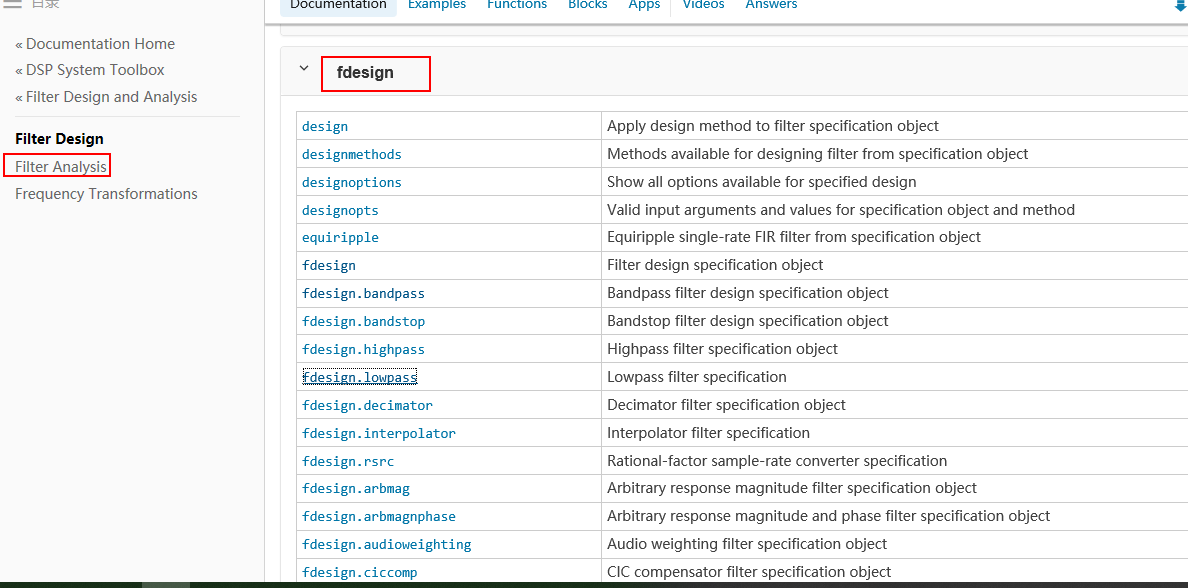简单设计了低通滤波

%% 低通滤波
function filtered = LowFilter(x)
fs_origin=1;
fc=0.03;
N=4;
hd_low = design(fdesign.lowpass('N,F3dB',N,fc, fs_origin),'butter');
filtered = filter(hd_low,x);
end

..


展开全文• 基于matlab函数的FIR带通滤波器设计.pdf
• 巴特沃斯带通滤波器这个简单的函数是为我的数字图像处理课程编写的在东地中海大学任教副. 哈桑·德米雷尔（Hasan Demirel）教授2010-2011 Spring学期完整报告： ...
• 滤波器类型的选择可根据滤波器设计的带宽...由上面的指标可以看出本滤波器是窄带带通滤波器。采用巴特沃斯滤波器来设计可以使通带内具有最大平坦的幅频响应；而切比雪夫滤波器的好处是：带外抑制好，但是带内有一定...
滤波器类型的选择可根据滤波器设计的带宽等指标和具体的应用场合来选择。相对带宽在20%以下的为窄带滤波器，应选用窄带滤波器的设计方法来设计；相对带宽在40%以上的为宽带滤波器，应选用宽带滤波器的设计方法来设计；而介于两者之间的为中等带宽滤波器。由上面的指标可以看出本滤波器是窄带带通滤波器。采用巴特沃斯滤波器来设计可以使通带内具有最大平坦的幅频响应；而切比雪夫滤波器的好处是：带外抑制好，但是带内有一定的波动；本文设计的滤波器要求带外近端抑制良好(可以用切比雪夫滤波器或椭圆函数滤波器来实现，但是从后面的分析看要使用LC滤波器，而用LC滤波器的话，使用切比雪夫形式电路元件的值过于小，很难实现，这个可以用软件仿真来说明)，以此可以看出，用椭圆函数滤波器更适合。微带滤波器通过采用不同的衬底材料可以在很大的频率范围内应用(从几百MHz到几十GHz)；同轴滤波器由于其微小的尺寸，制作精度很难达到；波导滤波器在小信号电平上，它的频率基本是8~100 GHz;陶瓷介质滤波器体积大，形状因子与品质因数较小；LC滤波器适用于本滤波器频段，且较容易制作和调试。综上，本设计采用：椭圆函数LC带通滤波器进行设计。设计指标如下：中心频率为450 MHz;工作带宽为20 MHz;带内插损为《3 dB;带外抑制为-40 dB.1 关于滤波器阶数N 的选择滤波器级数N 是一个重要的参数，它的选择直接影响到滤波器的性能，特别是滤波器的插损和带外特性，所以N 的选择非常重要。先对可能用到的一些概念和公式进行简单的说明。对于终端短路式滤波器其滤波器级数N 应满足下面关系式：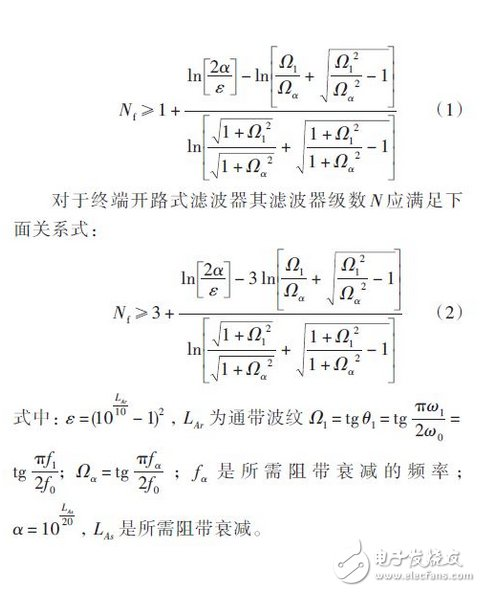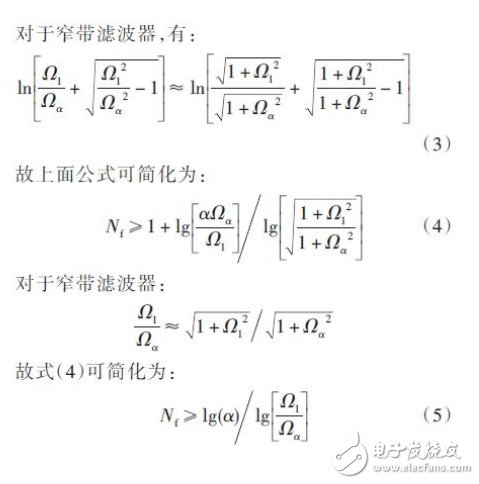对于带通滤波器，起相对带宽小于20%，所以其属于窄带滤波器。由上面的公式可以算出：N 必须大于或等于3，才能满足上面的近似指标。
展开全文• 模拟 2 个已知信号的带通滤波器（它不适用于随机信号）。 - 将信号转换为频域。 - 去除不需要的频率（即 6.5 Hz）。 - 再次将信号转换为时域。 所有模拟都在没有内置傅立叶传输的情况下完成。 感谢 Mike X Cohen ...
• 原始信号由5Hz，50Hz，110Hz三种频率的正弦信号构成...​使用通带为[10,100]Hz的切比雪夫滤波器，滤波后的信号时域曲线为： ﻿图 2 滤波信号 ​对原始信号和滤波信号作傅里叶变换，观察频谱的变化（左图为原始信号...
原始信号由5Hz，50Hz，110Hz三种频率的正弦信号构成，并含有直流分量。

原始信号为：y=sin(5*2*pi*x)+sin(50*2*pi*x)+sin(110*2*pi*x)+0.5;​﻿图 1   原始信号

​使用通带为[10,100]Hz的切比雪夫滤波器，滤波后的信号时域曲线为：﻿图 2   滤波信号

​对原始信号和滤波信号作傅里叶变换，观察频谱的变化（左图为原始信号频谱，有图为滤波信号频谱）。﻿图 3   频谱对比

​对比图 3 可见，原始信号5Hz，110Hz的交流成分和直流成分都被滤掉了，只保留了50Hz交流成分，并且幅值吻合。

附切比雪夫II型带通滤波器程序

​function [X,Y]=Chebyshev2bp(x,y,fp,fs,Rp,Rs)

% fp 通带边界频率，单位Hz

% fs 阻带边界频率，单位Hz

% Rp 通带波纹，单位分贝（通常取值为1，该值越小通带幅值越平稳，但过渡带宽也越大）

% Rs 阻带衰减，单位分贝（从30附近试取，该值越大阻带幅值越小，但过渡带宽也越大）

wp=fp*2*pi;

ws=fs*2*pi;

[N,Wn]=cheb2ord(wp,ws,Rp,Rs,'s');  % 根据滤波器要求，求得滤波器最小阶数（计算也越慢）和截止频率（万永革 例5-17）

N

%%% 关于滤波器阶数N的说明

% 理论上，滤波器阶数越高越接近理想滤波器，但滤波器阶数达到一定值后再增加阶数，其逼近程度并不再显著提高，但计算量依然增加，所以理论上不需要无穷大的阶数

% 实际编程中，随着阶数无限升高，更根本的问题是计算机积累误差凸显，将使其与理想滤波器相去甚远，最终时域幅值严重失真，所以实际应用中滤波器阶数也不是越高越好

[z,p,k]=cheb2ap(N,Rs);  %设计Chebyshev II型原型低通滤波器（万永革 例5-8）

[b,a]=zp2tf(z,p,k);  % 将零点极点增益形式转换为传递函数形式

Wo=sqrt(Wn(1)*Wn(2));  % 计算中心点频率（万永革 例5-14）

Bw=Wn(2)-Wn(1);  % 计算频带宽度

[bt,at]=lp2bp(b,a,Wo,Bw);  % 频率转换。将模拟原先低通滤波器转换为带通滤波器

H=[tf(bt,at)];  % 在MATLAB中表示此滤波器（万永革 例5-13）

[Y,X]=lsim(H,y,x);

https://www.cnblogs.com/alimy/p/9140695.html
展开全文切比雪夫
• 最近在处理数据过程中想着将数据进行一下滤波，所以就在查找资料同时记录一下学到的东西。在MATLAB中用带通滤波器滤波
• ## matlab设计模拟带通滤波器

万次阅读 多人点赞 2018-10-24 21:11:13
简单记录下在matlab上如何设计出模拟的带通滤波器，包括：巴特沃斯滤波器、切比雪夫I型滤波器、切比雪夫II型滤波器、椭圆型滤波器。 代码如下： %设计带通滤波器 %巴特沃斯、切比雪夫I型、切比雪夫II型、椭圆型...
• 带通滤波器——巴特沃斯滤波器为例matlab给出的代码如下：[n,Wn] = buttord(Wp,Ws,Rp,Rs)是不是显得很不人性化，即便是看了help中的英文解释，仍旧是对输入输出参数一知半解~那么我就用简单的语言来描述一下~首先...
• 摘 要：本文以70MHz带通滤波器为例，论述了如何采用BESSEL函数进行精确带通滤波器设计，同时利用PSPICE和MATLAB软件对设计结果进行波特图和群延迟特性仿真。关键词：BESSEL函数带通滤波器；PSPICE；MATLAB；群延迟...
• 武汉理工大学Matlab 课程设计报告 课程设计任务书 学生姓名 专业班级 通信 0705 指导教师 工作单位 信息工程学院 题 目:基于窗函数法设计的数字带通 FIR 滤波器 初始条件 MATLAB 软件 数字信号处理与图像处理基础...
• Matlab结合窗函数法设计数字带通FIR滤波器 课程设计任务书学生姓名： 专业班级： 通信工程 指导教师： 工作单位： 信息工程学院 题 目:利用 Matlab 仿真软件系统结合窗函数法设计一个数字带通 FIR 滤波器初始条件： ...
• cornerFreqs 自动检测合适的带通滤波器角点通过将输入信号的频谱与噪声频谱进行比较来确定频率。 动机： 地震波形的处理通常需要带通滤波。 滤波器转角频率的选择不仅仅是手动操作过程也是主观的。 需要自动检测角...
• 基于Matlab GUI的模拟带通滤波器的设计、电子技术,开发板制作交流代码优化
• 如何在matlab带通滤波器进行滤波？ matlab中fdatool使用说明 Matlab fir滤波(conv) 以上链接详细记录了带通滤波器的设计及matlab自带fdatool的使用步骤。 自己编的一个带通滤波器的程序及解释 fs=500; % ...
• 基于MATLAB的FIR带通滤波器的设计与仿真 摘要利用数字信号处理理论与方法基于MATLAB语言通过实例设计FIR数字带通滤波器给出了MATLAB完整程序实例仿真结果表明该带通滤波器的滤波效果达到了预期目的 关键词数字滤波器...
• matlab代码，直接运行出结果。FIR带通滤波器，并对数字信号进行带通滤波。建立含有3种频率和白噪声的信号。然后利用窗函数法设计
• ## 死磕带通滤波器

千次阅读 2021-04-24 20:51:17
带通滤波器的作用 ...带通滤波器传递函数 带通滤波器的传递函数是： h(s)=AwoBss2+Bs+wo2 h(s)=\frac{Aw_oBs}{s^2+Bs+w_o^2} h(s)=s2+Bs+wo2​Awo​Bs​ 其中，wow_owo​ 是带通的“中心频率”，也就是想要通过频率
• 　模拟带通滤波器在信号的检测和传输过程中起着很重要的作用，但在传统模拟带通滤波器的设计过程中需要大量繁琐的数值计算，如果手工计算则费时费力。也有许多公司开发了相关的滤波器设计软件，如AnsoftDesigner，...
• 巴特沃斯带通滤波器matlab代码肌电图 注意：这是我多年未曾使用过的学校项目中的旧代码，太幼稚了。 使用面向对象的方法会更好。 信号处理位是声音，因此请使用它们作为灵感，但我不建议在此使用任何其他功能。 ...
• matlab开发-用于图像处理的高斯带通滤波器。此函数在频域中执行高斯带通滤波。
• 该EXCEL表格可用于辅助带通滤波器设计。用户输入带通滤波器性能指标，表格快速给出低通原型滤波器的元件参数以及带通滤波器的归一化耦合系数、输入群时延、外部Q值等
• Matlab通过创建应用程序M文件为GUI控制程序提供一个框架。所有代码(包括回调函数)都包含在应用程序M文件中，即完成了图形界面的设计后，应用程序设计的主要工作是控件回调函数的设计。下面讨论程序设计流程及控件回...
• 产生信道高斯白噪声，设计信道带通滤波器对高斯白噪声进行滤波，得到窄带高斯噪声。对信道带通滤波器的输入输出的噪声的时域、频域特性进行统计和分析，画出其时域和频域的图形。 高斯白噪声产生 首先确定采样频率和...
• 二阶巴特沃斯带通滤波器仿真电路图，二阶巴特沃斯带通滤波器仿真电路图...

# matlab带通滤波器函数matlab 订阅